Home  │ Audio Home Page

Updated January 2, 2013.  See Document History at end for details.

# DAC Cascode

Analog DAC circuit designed with one BJT

### Introduction

Current to voltage conversion is commonly needed to convert the output of many DACs.  For any who requires a single transistor converter rather than the usual op-amp I/V converter, the BJT cascode is most obvious candidate.

### PNP Circuit

 Figure 1:  Schematic of single-ended analog circuit..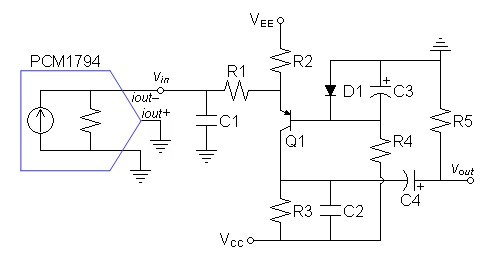Note: Only left channel shown.  Duplicate for the right channel.

The circuit of figure 1 above adds several features to the ordinary BJT cascode of Q1.  R2 increases the bias through Q1 to lower overall distortion.  D1 biases the emitter of Q1 to ≈ 0V.  C3 removes  nonlinearities from drive to Q1 base for the calculated range of higher frequencies and prevent RF from intermodulating in D1.  C1 and C2 create two synchronous lowpass poles with respect to their related resistances to give an overall cutoff frequency of 100kHz.  C1 in particular is positioned before Q1 to reduce intermodulation with DAC RF frequencies.  R1 is added to reduce variations in AC emitter impedance related to signal level which tend to create a changing pole frequency with respect to C1.  As for the unused diffential DAC output, it is reasonable to connect it separately to the same voltage that the other sinks into.

This design for the PCM1794 will also work for the comparable PCM1792 also.  For other current output DACs as the other PCM179x (the PCM1795, PCM1796, and PCM 1798), it would be necessary to recalculate for the different current output specifications.  If the DAC sinks current rather than sources it as these do, the circuit polarity could be flipped and an NPN transistor used instead of a PNP.  The AD1955 is one of these, not only requiring a NPN design but slightly different biasing as well.

### First Try with PCM1794

I set R3 and R5 to the same value in the first attempt thinking R3 could drive an equal resistance in R5 with R5 perhaps off the circuit board at the output jack.  Placing R5 at the end of dedicated interconnects could even eliminate cable effects.

#### Evaluate Datasheet

The datasheet for the PCM1794 gives the following output specifications:
 AC output 7.8mA peak-to-peak for each single-ended output (I use the 3.9mA peak value in the calculations) DC offset –6.2mA Output impedance Unspecified

Because the DAC topology seems to source current rather than sink it the negative current value seemed unintuitive to me, I expected it to be positive.  As a result, I examined the remainder of the datasheet for other clues.  The ones I found were:
• The recommended analog circuits show output driving virtual ground at operational amplifier.
• DAC has only positive voltages to drive the 0V virtual ground.
• Digital output current specifications are consistent with attributing positive current polarity with current sinking.
Other datasheets I have examined seem to attribute positive current polarity to current sourcing, so examine your datasheets and recommended circuits carefully for consistency.

As for polarity, if an op-amp i/v converter could be considered inverting, the BJT cascode is non-inverting by comparison with regard to the AC signal.  Therefore the DACs polarity designations should be interpreted as reversed:  the –iout will actually be +iout.  As always examine the example analog circuit in the datasheet  to verify this.

#### Design

Begin by calculating two synchronous pole frequencies which together will roll off at 100kHz:
 (1) α  =
 2    2 – 1
 = 0.643594

 (2) fstage = fsystem α = 100kHz 0.643594 = 155.377kHz

Choose VEE = 15V
Choose VCC = –30V to give room for desired bias.
Calculate R3 and R5 to convert 3.9mA input signal to 2VRMS output signal
 (3) R3 = 2 × 1.41421 × 2V 3.9mA = 1.45048kΩ
Round down to nearest 1% value:
R3 and R5 = 1.43kΩ

Calculate R2 for 5V headroom below ground:
 (4) IQ1 = 30V – 5V 1.43kΩ = 17.4825mA

 (5) IR2 = 17.4825mA – 6.2mA – 1.95mA = 9.33252mA

 (6) R2 = 15V 9.33252mA = 1.60728kΩ
Round to nearest 5% value:
R2 = 1.6kΩ

Calculate C2 for second synchronous lowpass pole:
 (7) C2 = 1 2πfpoleRPOLE = 1 2π × 155.377kHz × (1.43kΩ/2) = 1.43261nF
Round down to nearest 5% value:
C2 = 1.3nF

To prepare to calculate for first lowpass pole, calculate an R1 value to add with the AC emitter impedance to total about 10Ω.
First calculate minimum emitter current.
 (8) IQ1-MIN = 15 1.6kΩ + 6.2mA – 3.9mA = 11.675mA

Then calculate maximum emitter impedance:
 (9) ZQ1E-MIN = 25mV 11.675mA = 2.14133Ω

Then calculate R1.
 (10) R1 = 10Ω – 2.14133Ω = 7.85867Ω
Decide to round up to nearest 5% value:
R1 = 8.2Ω

Now C1 can be calculated.
 (11) C1 = 1 2πfpoleRPOLE = 1 2π × 155.377kHz × (2.14133Ω + 8.2Ω) = 99.0506nF
Round to nearest 5% value:
C1 = 100nF

Calculate 1W R4 to dissipate ½W:
 (12) R4 = V2 P = (30V – 0.7V)2 0.5 = 1.71698kΩ
Round to nearest 5% value:
R4 = 1.8kΩ

Initially I arbitrarily chose C3 = 47µF but decided to calculate for a subsonic pole, however the 750µF I calculated against the Q1 base input impedance should have instead have been calculated against the ac impedance of D1.  In that case, a subsonic pole could not be achieved without an impractically enormous value for C3.  Until now leave as is.

Calculate C4 for a time constant of 1s.
 (13) C4 = T RPOLE = 1s 1.43kΩ + 1.43kΩ = 349.6503497µF
Round to nearest 10% value:
C4 = 330µF

 Parts List Q1 2N3906 PNP R5 1.43kΩ 1% ½W or 1W D1 1N914 or 1N4148 C1 100nF 5% plastic R1 8.2Ω 5% ¼W C2 1.3nF 5% plastic R2 1.6kΩ % ½W C3 47µF 10% aluminum electrolytic R3 1.43kΩ 1% 1W C4 330µF 10% aluminum electrolytic R4 1.8kΩ 5% 1W

Prefer to upgrade Q1 to a small-signal PNP in T0-5 package (metal can).
C1 and C2 are preferred in this order: polystyrene, teflon, polypropylene, …

#### SPICE Analysis

Correction

The spice models for this and the next analysis were incorrect on the following line:

idac 0 vin dc 6.2m ac 1 sin 0 3.9m 1kHz

The error here is that the dc current offset after the dc keyword does not count for the transient analysis, it has to be present in the sin statement as well.

I corrected the line to:

idac 0 vin dc 6.2m ac 1 sin 6.2m 3.9m 1kHz

and reran both analyses.  Only the distortion results were changed.

SPICE model

 Figure 2:  Bode plot shows cutoff frequency slightly lower than 100kHz goal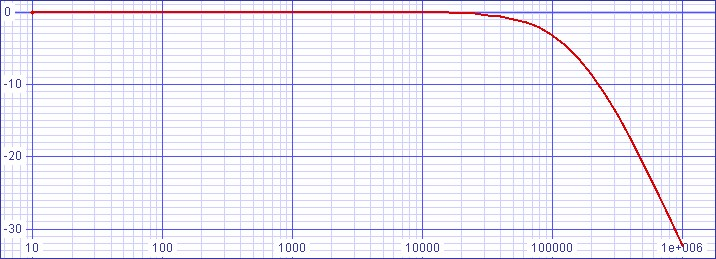Distortion results are primarily second and third harmonic

Fourier analysis for vout:
No. Harmonics: 16, THD: 0.0420128 %, Gridsize: 1024, Interpolation Degree: 3

 Harmonic Frequency Magnitude Norm.Mag Percent Decibels 1 1000 2.69196 1 100 0 2 2000 0.00111459 0.000414043 0.0414043 -67.65909107 3 3000 0.000191641 7.11902E-05 0.00711902 -82.95159574 4 4000 4.70198E-06 1.74667E-06 0.000174667 -115.1557828 5 5000 2.86806E-06 1.06542E-06 0.000106542 -119.4495831 6 6000 9.57495E-07 3.55687E-07 3.55687E-05 -128.9786402 7 7000 2.37009E-06 8.8043E-07 0.000088043 -121.1061034 8 8000 1.10266E-06 4.09612E-07 4.09612E-05 -127.7525466 9 9000 2.13659E-06 7.93694E-07 7.93694E-05 -122.0069381 10 10000 1.07911E-06 4.00865E-07 4.00865E-05 -127.9400372 11 11000 1.93559E-06 7.19026E-07 7.19026E-05 -122.8651081 12 12000 4.9167E-07 1.82644E-07 1.82644E-05 -134.7678918 13 13000 1.9412E-06 7.21108E-07 7.21108E-05 -122.8399937 14 14000 2.00793E-07 7.459E-08 0.000007459 -142.5463879 15 15000 1.45375E-06 5.40032E-07 5.40032E-05 -125.3516101

Out of curiosity, without extra current bias to Q1 from R2, distortion is 0.199071%.

Signal to noise ratio:
119.6568dB relative to 1VRMS.

Relative to 2.6952VPEAK/1.90579VRMS at full-scale level add 5.60152dB for
125.258dB relative to 0dBFS.

### Attempt at an Improvement

In my second try, setting R5 to a very high value to allow R3 to take a smallest possible value promises to lower distortion by allowing a design with higher bias through Q1.

#### Design

The two synchronous pole frequencies will be 155.377kHz as they were calculated in equations 1 and 2 above.

Choose VEE = 15V.
Choose VCC = –30V to give room for desired bias.
Calculate R3 and R5 to convert 3.9mA input signal to 2VRMS output signal.

 (14) R3 || R5 = 1.41421 × 2V 3.9mA = 725.238Ω

Choose
R5 = 100kΩ.
Subtracting
R5 from the parallel pair R3 || R5 leaves R3.
 (15) R3 = 100kΩ × 725.238Ω 100kΩ – 725.238Ω = 730.536Ω
Round down to nearest 1% value:
R3  = 732Ω

Calculate R2 for 5V headroom below ground:
 (16) IQ1 = 30V – 5V 732Ω = 34.153mA

 (17) IR2 = 34.153mA – 6.2mA – 3.9mA = 24.053mA

 (18) R2 = 15V 24.053mA = 623.623Ω
Round to nearest 5% value:
R2 = 620Ω

Calculate C2 for second synchronous lowpass pole:
 (19) C2 = 1 2πfpoleRPOLE = 1 2π × 155.377kHz × (732Ω || 100kΩ) = 1.40958nF
Round down to nearest 5% value:
C2 = 1.3nF

To prepare to calculate for first lowpass pole, calculate an R1 value to add with the AC emitter impedance to total about 10Ω.
First calculate minimum emitter current.
 (20) IQ1-MIN = 15 620Ω + 6.2mA – 3.9mA = 26.4935mA

Then calculate maximum emitter impedance:
 (21) ZQ1E-MIN = 25mA 26.4935mA = 943.626mΩ

Then calculate R1.
 (22) R1 = 10Ω – 943.626mΩ = 9.05637Ω
Decide to round up to nearest 5% value:
R1 = 9.1Ω

Now C1 can be calculated.
 (23) C1 = 1 2πfpoleRPOLE = 1 2π × 155.377kHz × (943.626mΩ + 9.1Ω) = 101.987nF
Round to nearest 5% value:
C1 = 100nF

Calculate 1W R4 to dissipate ½W:
 (24) R4 = V2 P = (30V – 0.7V)2 0.5 = 1.71698kΩ
Round to nearest 5% value:
R4 = 1.8kΩ

Initially I arbitrarily choose C3 = 47µF

Calculate C4 for a time constant of 1s.
 (25) C4 = T RPOLE = 1s 732Ω + 100kΩ = 9.92733µF
Round to nearest 10% value:
C4 = 10µF

After some thought, I realized that loading would affect the calculation of C4 here.  In the first circuit, a 10kΩ or 47kΩ load would appear insignificant in parallel with 1.43kΩ.  For that reason, I originally disregarded loading there.  However, here
47kΩ lowers the R5 || RL combination by more than a factor of 3.  Even still, raising a calculated pole from 0.1592Hz to 0.4978Hz still may not merit an alteration to the calculated value if only a 47kΩ load is expected.  However for completeness, recalculating with a 47kΩ load will give a new value for C4:  30.5766µF.

Round to nearest 10% value:
C4 = 30µF

 Parts List Q1 2N3906 PNP R5 100kΩ 5% ¼W or ½W D1 1N914 or 1N4148 C1 100nF 5% plastic R1 9.1Ω 5% ¼W C2 1.3nF 5% plastic R2 620Ω % ½W C3 47µF 10% aluminum electrolytic R3 732Ω 1% 2W C4 30µF 10% plastic R4 1.8kΩ 5% 1W

Prefer to upgrade Q1 to a small-signal PNP in T0-5 package (metal can).
C1 and C2 are preferred in this order: polystyrene, teflon, polypropylene, …
C4 is preferred in this order: polypropylene, polycarbonate, polyester (Mylar), …

#### SPICE Analysis

SPICE model  of improved circuit.

 Figure 3:  Bode plot still shows cutoff frequency slightly lower than 100kHz goal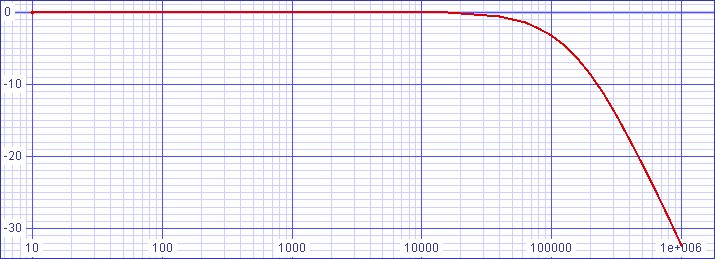Distortion results are primarily second and third harmonic
Fourier analysis for vout:
No. Harmonics: 16, THD: 0.108487 %, Gridsize: 1024, Interpolation Degree: 3

 Harmonic Frequency Magnitude Norm.Mag Percent Decibels 1 1000 2.71265 1 100 0 2 2000 0.00293561 0.00108219 0.108219 -59.31392967 3 3000 0.000206089 7.59733E-05 0.00759733 -82.38678018 4 4000 0.000010125 3.7325E-06 0.00037325 -108.5600037 5 5000 4.86714E-06 1.79424E-06 0.000179424 -114.9223893 6 6000 6.40395E-08 2.36078E-08 2.36078E-06 -152.5388897 7 7000 5.0589E-06 1.86493E-06 0.000186493 -114.5867493 8 8000 6.24975E-08 2.30393E-08 2.30393E-06 -152.7506144 9 9000 4.0545E-06 1.49467E-06 0.000149467 -116.5090936 10 10000 4.22788E-07 1.55858E-07 1.55858E-05 -136.145418 11 11000 2.47042E-06 9.10705E-07 9.10705E-05 -120.8124456 12 12000 3.31809E-07 1.22319E-07 1.22319E-05 -138.2501216 13 13000 1.05783E-06 3.89963E-07 3.89963E-05 -128.1795319 14 14000 6.45024E-07 2.37784E-07 2.37784E-05 -132.4763474 15 15000 4.6302E-07 1.70689E-07 1.70689E-05 -135.3558893

Signal to noise ratio:
117.8938dB relative to 1VRMS.

Relative to 2.72627VPEAK/1.92776VRMS at full-scale level add 5.70108dB for
123.595dB relative to 0dBFS.

#### Result of Change

Paradoxically, the improvement has increased the distortion.  I believe this result is due to distortion having some inversely proportional relationship to the value of R2 as well at to the current bias change.  Because primarily the second harmonic was raised leaving the third harmonic the same this change becomes a more euphonic preference.  Perhaps even more important the change allows the use of a lower distortion capacitor at C4.  The second circuit is not recommended to drive loads lower than 47kΩ.

### Design an NPN circuit

 Figure 4:  Schematic of single-ended analog circuit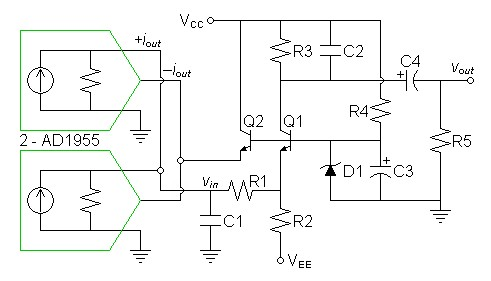Note: Only left channel shown.  Duplicate for the right channel.

This NPN circuit is only flipped in polarity from the PNP circuit first introduced apart from two details:  That this circuit is biased with a zener rather than an normal diode because the particular DAC requires its current output to sink into a non-zero voltage and the decision to use two DACs.

#### Evaluate Datasheet

The datasheet for the AD1955 gives the following output specifications:
 AC output 8.64mA peak-to-peak differential 2.16mA peak single ended DC offset –3.24mA (specified as current sink) Output impedance Unspecified Analog sink voltage 2.80V ideal, down to low of 2.39V

Analyzing polarity of reference analog circuit suggests no polarity reversal for connection.  Because the output current specifications are expected to design to a high output impedance, use two parallel devices.

As a parallel pair their specifications are now:
 AC output 17.28mA peak-to-peak differential 4.32mA peak single ended DC offset -6.48mA (specified as current sink) Output impedance Unspecified Analog sink voltage 2.80V ideal, down to low of 2.39V

#### Design

Begin by calculating two synchronous pole frequencies which together will roll off at 100kHz:
 (26) α =
 2   2 – 1
 = 0.643594

 (27) fstage = fsystem α = 100kHz 0.643594 = 155.377kHz

Choose VEE = –15V
Choose VCC = 30V to give room for desired bias.
Assume rated output current is dropped between the optimal output sink voltage and ground.  Then calculate DAC output impedance:
 (28) RDAC = VSINK – GND IDAC-MAX = 2.8V - 0V 6.48mA + 4.32mA = 259.259Ω
Note:  This DAC has no R-2R resistor ladder determining its output current that any certainty could be attributed to this output impedance.  It could have open collector/drain outputs instead of resistive.  However removing RDAC in the simulation does not seem to significantly alter the final simulation results.

Calculate D1 zener voltage
 (29) Vzener-D1 = 2.8V + 0.7V = 3.5V
Round down to nearest 5% value to bracket new sink voltage to 2.39V–2.8V range.
Vzener-D1 = 3.3V
Operating sink voltage will now be:
.VSINK = 2.6V

Calculate R3 and R5 to convert 3.9mA input signal to 2VRMS output signal
 (30) R3 = 2 × 1.41421 × 2V 4.32mA = 1.30946kΩ
Round up to nearest 1% value:
R3 and R5 = 1.33kΩ
Here, I decided to round up to compensate for the slight loss of signal current to the current divider created by RDAC and R1.

Calculate R2 for 5V headroom below ground:
 (31) IQ1 = 30V - 5V - 3.3V 1.33kΩ = 16.3158mA

 (32) IR2 = 16.3158mA - 6.48mA - 2.16mA = 7.67579mA

 (33) R2 = 15V + 2.6V 7.67579mA = 2.29292kΩ
Round up to nearest 5% value:
R2 = 2.4kΩ

Calculate C2 for second synchronous lowpass pole:
 (34) C2 = 1 2πfpoleRPOLE = 1 2π × 155.377kHz × (1.33kΩ/2) = 1.54032nF
Round down to nearest 5% value:
C2 = 1.5nF

To prepare to calculate for first lowpass pole, calculate an R1 value to add with the AC emitter impedance to total about 10Ω.
First calculate minimum emitter current.
 (35) IQ1-MIN = 15V + 2.6V 2.4kΩ + 6.48mA - 4.32mA = 9.49333mA

Then calculate maximum emitter impedance:
 (36) ZQ1E-MIN = 25mV 9.49333mA = 2.63343Ω

Then calculate R1.
 (37) R1 = 10Ω – 2.63343Ω = 7.36657Ω
Decide to round up to nearest 5% value:
R1 = 7.5Ω

Now C1 can be calculated.
 (38) C1 = 1 2πfpoleRPOLE = 1 2π × 155.377kHz × (2.63343Ω + 7.5Ω) = 101.083nF
Round to nearest 5% value:
C1 = 100nF

Calculate 1W R4 to dissipate ½W:
 (39) R4 = V2 P = (30V – 3.3V)2 0.5 = 1.42578kΩ
Round up to nearest 5% value:
R4 = 1.5kΩ

Arbitrarily chose C3 = 47µF.

Calculate C4 for a time constant of 1s.
 (40) C4 = T RPOLE = 1s 1.33kΩ + 1.33kΩ = 375.94µF
Round to nearest 10% value:
C4 = 390µF

 Parts List Q1,Q1 2N3904 PNP R5 1.33kΩ 1% ½W or 1W D1 3.3V zener C1 100nF 5% plastic R1 7.5Ω 5% ¼W C2 1.5nF 5% plastic R2 2.4kΩ % ½W C3 47µF 10% aluminum electrolytic R3 1.33kΩ 1% 1W C4 390µF 10% aluminum electrolytic R4 1.5kΩ 5% 1W

Prefer to upgrade Q1 to a small-signal NPN in T0-5 package (metal can).
C1 and C2 are preferred in this order: polystyrene, teflon, polypropylene, …

#### SPICE Analysis

SPICE model

 Figure 5:  Bode plot still shows cutoff frequency slightly lower than 100kHz goal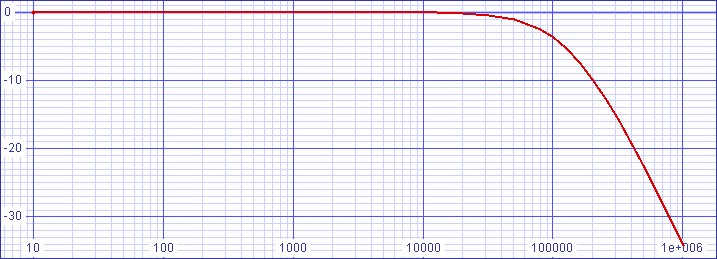Fourier analysis for vout:
No. Harmonics: 16, THD: 0.0415427 %, Gridsize: 1024, Interpolation Degree: 3

 Harmonic Frequency Magnitude Norm. Mag Percent Decibels 1 1000 2.847 1 100 0 2 2000 0.00117564 0.000412941 0.0412941 -67.6822 3 3000 0.000128299 4.50648E-05 0.00450648 -86.9233 4 4000 1.39636E-05 4.90466E-06 0.000490466 -106.188 5 5000 4.21864E-06 1.48179E-06 0.000148179 -116.584 6 6000 5.05001E-07 1.7738E-07 0.000017738 -135.022 7 7000 2.64547E-06 9.29215E-07 9.29215E-05 -120.638 8 8000 1.00591E-06 3.53324E-07 3.53324E-05 -129.037 9 9000 1.88327E-06 6.61494E-07 6.61494E-05 -123.589 10 10000 7.95153E-07 2.79295E-07 2.79295E-05 -131.079 11 11000 1.15167E-06 4.0452E-07 0.000040452 -127.861 12 12000 1.5341E-07 5.38848E-08 5.38848E-06 -145.371 13 13000 1.31741E-06 4.62736E-07 4.62736E-05 -126.693 14 14000 2.86087E-07 1.00487E-07 1.00487E-05 -139.958 15 15000 1.11035E-06 3.90009E-07 3.90009E-05 -128.179

Signal to noise ratio:
122.0053dB relative to 1VRMS.

Relative to 2.847VPEAK/2.01313VRMS at full-scale level add 6.07745dB for
128.083dB relative to 0dBFS.

Document History
December 1, 2012  Created.
December 1, 2012  Added equation numbers to design
and some extra text and rescinded 750µF calculation for C3.
December 1, 2012  Added a second design sequence aimed to improve distortion.
December 2, 2012  Added text recalculating a new C4 value for the second circuit under load.
December 4, 2012  Corrected 25mA in equation 9 to 25mV.
December 7, 2012  Correcting SPICE model error changed distortion results somewhat.  Reworded statement about useful DACs to indicate that the AD1955 will require an NPN design.  Added paragraph on the interpretation of the datasheet.
January 1, 2013  Added an NPN design and analysis based on the AD1955.
January 2, 2013  Changed schematics to show how unused DAC outputs should be terminated.